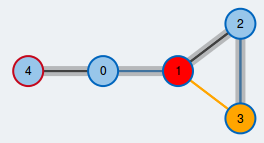Edmonds's Blossom Algorithm# Maximum matchings in general graphs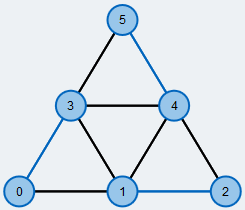Maximum matching in a general graph.
Edges contained in the matching are colored blue.

An often occuring and well-studied problem in graph theory is finding a maximum matching in a graph $$G=(V,E)$$. A matching M is a subset of edges such that every node is covered by at most one edge of the matching. M is a maximum matching if there is no other matching in G that has more edges than M.

This website is about Edmonds's Blossom Algorithm, an algorithm that computes a maximum matching in an undirected graph. In contrast to some other matching algorithms, the graph need not be bipartite. The algorithm was introduced by Jack Edmonds in 1965 and has been further improved since then. Many exact modern algorithms for the maximum matching problem on general graphs are still based on the ideas of the Blossom Algorithm.

Edmonds's Blossom Algorithm uses and extends the essential ideas of the Hopcroft-Karp algorithm, which computes a maximum matching for bipartite graphs. If you have not heard about this algorithm, we recommend having a look at it before proceeding with the Blossom Algorithm: Hopcroft-Karp Algorithm

We further assume that you are familiar with graph traversal, especially Breadth-First Search.

## Legendnode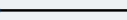undirected edge

## Which graph do you want to execute the algorithm on?

### Modify it to your desire:

• To create a node, make a double-click in the drawing area.
• To create an edge, first click on the output node and then click on the destination node.
• Right-clicking deletes edges and nodes.

A occured when reading from file: the contents:

## Legend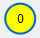yellow: free node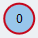red stroke: root of the BFSred: currently active node in BFSorange: currently checked neighbor in BFS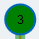green: node on the augmenting path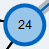large radius: contracted node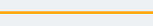orange: currently checked edge in BFS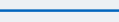blue: matched edge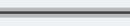grey overlay: edge in BFS treegreen overlay: edge on the augmenting path

## Algorithm status

### Edmonds's Blossom Algorithm

The algorithm is ready to start now. Click "next" to execute a single step of the algorithm, "prev" to go one step back and "fast forward" for a fast execution of the algorithm.

The BFS queue is empty. No augmenting path has been found by the modified Breadth-First Search.

### Improved matching

The augmenting path is inverted to improve the matching. Inverting an augmenting path means that all unmatched edges along the path are changed to matched ones and vice versa. By doing so, the number of edges contained in the matching increases by 1. The new matching is colored blue.

### Free vertices left

There are still unmatched vertices (yellow). We call them free vertices. We try to find an augmenting path to improve the matching. An augmenting path starts and ends at a free vertex and alternates between unmatched and matched edges.

We pick one of the free vertices as the root node of a modified Breadth-First Search (BFS). Its stroke is colored red throughout the execution of the BFS. From the root node, we will construct a tree of alternating paths (BFS tree) until we reach another free vertex.

### Pick next node from BFS queue

There are no neighbors left that we could check from the currently active node so we continue the BFS with the next node.

### Contract blossom

The odd-length cycle we found is contracted to a single supernode. Supernodes are highlighted by a larger radius compared to the other nodes. We further push the new supernode to the BFS queue.

### Continue BFS with next node

Pick the next node from the BFS queue. We call this node the currently active node and color it red. From this node, we will explore its neighbors to look for a free vertex.

### Grow BFS tree

We add the neighbor and its mate to the BFS tree. The mate is further pushed to the BFS queue and we might continue our search from there later. The edges of the BFS tree are highlighted by a grey overlay.

### Ignore even-length cycle

We can ignore even-length cycles. There is nothing to do in this case.

### Check next neighbor

We check the next neighbor (orange) and find that it is already matched.

### Check next neighbor

We check the next neighbor (orange edge) and find that it is a free vertex (yellow). Thus, there is an augmenting path between the root of the BFS and this vertex.

### Check next neighbor

We check the next neighbor (orange) and find that this node is already contained in the BFS tree. Thus, there exists a cycle and we see that it has an odd number of edges. We call such a cycle a blossom.

### Check next neighbor

We check the next neighbor (orange) and find that this node is already contained in the BFS tree. Thus, there exists a cycle and we see that it has an even number of edges.

### Reconstruction of augmenting path

The augmenting path is highlighted green. Before improving the matching we have to expand the supernodes contained in the graph.

### Expand supernodes

One of the supernodes is expanded and the augmenting path is adjusted correctly. There are still supernodes left that have to be expanded.

### Reconstruction of augmenting path

The augmenting path from the BFS root to the free vertex found has been fully reconstructed and is highlighted green.

### Reconstruction of augmenting path finished

After the expansion of all supernodes the augmenting path is fully reconstructed and highlighted green.

### Algorithm finished

There are no free vertices in the graph anymore so we have found a matching of maximum cardinality. Its edges are colored blue.

BEGIN

WHILE F != ∅ DO (*set of free nodes F*)

pick r ∈ F

queue.push(r) (*BFS queue*)

T ← ∅ (*BFS tree T*)

WHILE queue != ∅

v ← queue.pop()

FOR ALL neighbors w of v DO

IF w ∉ T AND w matched THEN

queue.push(mate(w))

ELSE IF w ∈ T AND even-length

cycle detected THEN

CONTINUE

ELSE IF w ∈ T AND odd-length

cycle detected THEN

contract cycle

ELSE IF w ∈ F THEN

expand all contracted nodes

reconstruct augmenting path

invert augmenting path

END

## If you switch tabs, the execution will be terminated.

You can open another browser window to read the description in parallel.